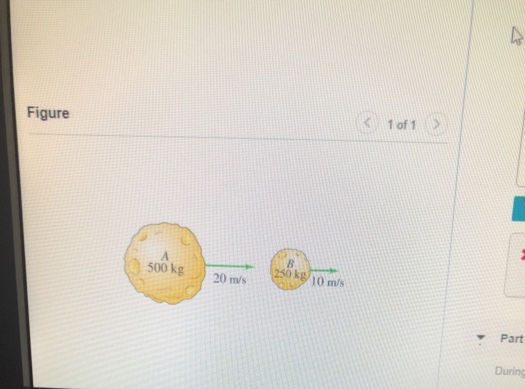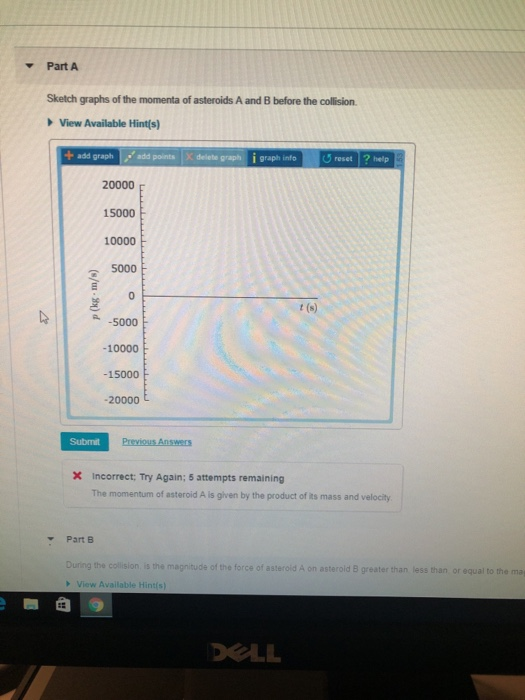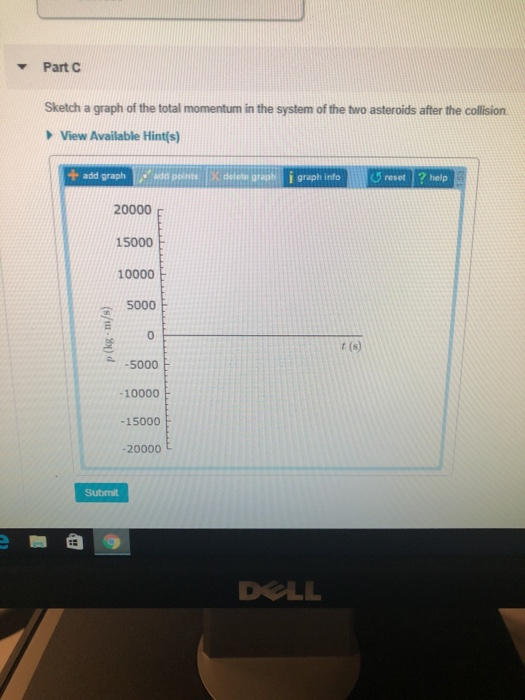# Problem: Two asteroids drifting at constant velocity collide. The masses and velocities of the asteroids before collision are indicated on the figurePart A Sketch graphs of the momenta of asteroids A and B before the collision.Part B During the collision, is the magnitude of the force of asteroid A greater than, less than or equal to asterold B?Part C Sketch a graph of the total momentum in the system of the two asteroids after the collision.

###### FREE Expert Solution

From the law of conservation of linear momentum, the total momentum before the collision is equal to the total momentum after the collision.

Momentum:

$\overline{){\mathbf{P}}{\mathbf{=}}{\mathbf{m}}{\mathbf{v}}}$

Part A

The momentum of asteroid A:

PA = (500)(20) = 10000 kg•m/s

91% (180 ratings)###### Problem Details

Two asteroids drifting at constant velocity collide. The masses and velocities of the asteroids before collision are indicated on the figurePart A Sketch graphs of the momenta of asteroids A and B before the collision.Part B During the collision, is the magnitude of the force of asteroid A greater than, less than or equal to asterold B?

Part C Sketch a graph of the total momentum in the system of the two asteroids after the collision.